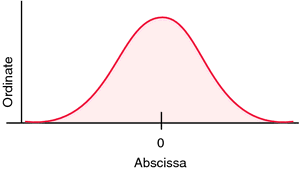# abscissa

Also found in: Dictionary, Thesaurus, Encyclopedia, Wikipedia.
Related to abscissa: Abscissa of convergence

## abscissa

[ab-sis´ah]
the horizontal line in a graph along which are plotted the units of one of the variables considered in the study, as time in a time-temperature study. The other line is called the ordinate.Axes of graph showing normal distribution curve.
Miller-Keane Encyclopedia and Dictionary of Medicine, Nursing, and Allied Health, Seventh Edition. © 2003 by Saunders, an imprint of Elsevier, Inc. All rights reserved.

## ab·scis·sa

(ab-sis'ă),
In a plane cartesian coordinate system, the horizontal axis (x). Compare: ordinate.
[L. ab-scindo, pp. -scissus, to cut away from]
Farlex Partner Medical Dictionary © Farlex 2012

## ab·scis·sa

(ab-sis'ă)
In a plane cartesian coordinate system, the horizontal axis (x).
[L. ab-scindo, pp. -scissus, to cut away from]
Medical Dictionary for the Dental Professions © Farlex 2012
Mentioned in ?
References in periodicals archive ?
Caption: Figure 2--Relationship between the Coefficient of Variation (%) for the weight of the medium cormels and plot size in Basic Units, to determine the abscissa value at the maximum curvature point ([X.sub.o]).
For both panels: on the ordinate, spinal NO metabolite concentration in [micro]mol/mg of tissue; on the abscissa, experimental groups.
Tmax (K) in May at Carbondale, with GCM on the abscissa and OBS on the ordinate, for historical snow-free (black), historical snow-covered (red), and future snow-free (orange) days.
The combined abscissa is calculated as a weighted average of the two extreme points with f(x) as the weights.
Figure 8 shows the relation between the average velocity of single outlet and applied electric field strength, where the ordinate is the average speed at a single exit v (mm/s) and the abscissa is the voltage at the power source U (V).
By default, the abscissa axis reports the apparent distance ([d.sup.app]); however, through an appropriate calibration, the actual physical distance (d) can be displayed.
(The abscissa axis shows frequency in Hz and the ordinate axis shows relative units).
In the log-log coordinate system, a groupn of dimensionless pressure type curves (Figure 2) are prepared, with [p.sub.D]([t.sub.D][e.sup.2S]) as ordinate against [t.sub.D][e.sup.2S] as abscissa and [b.sub.D][e.sup.-2S] as the curve parameter.
Linear regression analysis of 2B9.3 assay (ordinate) against S22(T) assay (abscissa).
In this contour plot, time is plotted as the ordinate, and wavelength is plotted as the abscissa, with the contour lines showing the amount of measured absorbance.
[c.sub.s]].sup.T] as the abscissa vector, and the vectors f([Y.sup.[n]]) = [[f([Y.sup.[n]i])].sup.s.sub.i=1] and g([Y.sup.[n]]) = [[g([Y.sup.[n].sub.i])].sup.s.sub.i=1] denote the first and second derivative stage values, where g(*) = f'(*)f(*).

Site: Follow: Share:
Open / Close